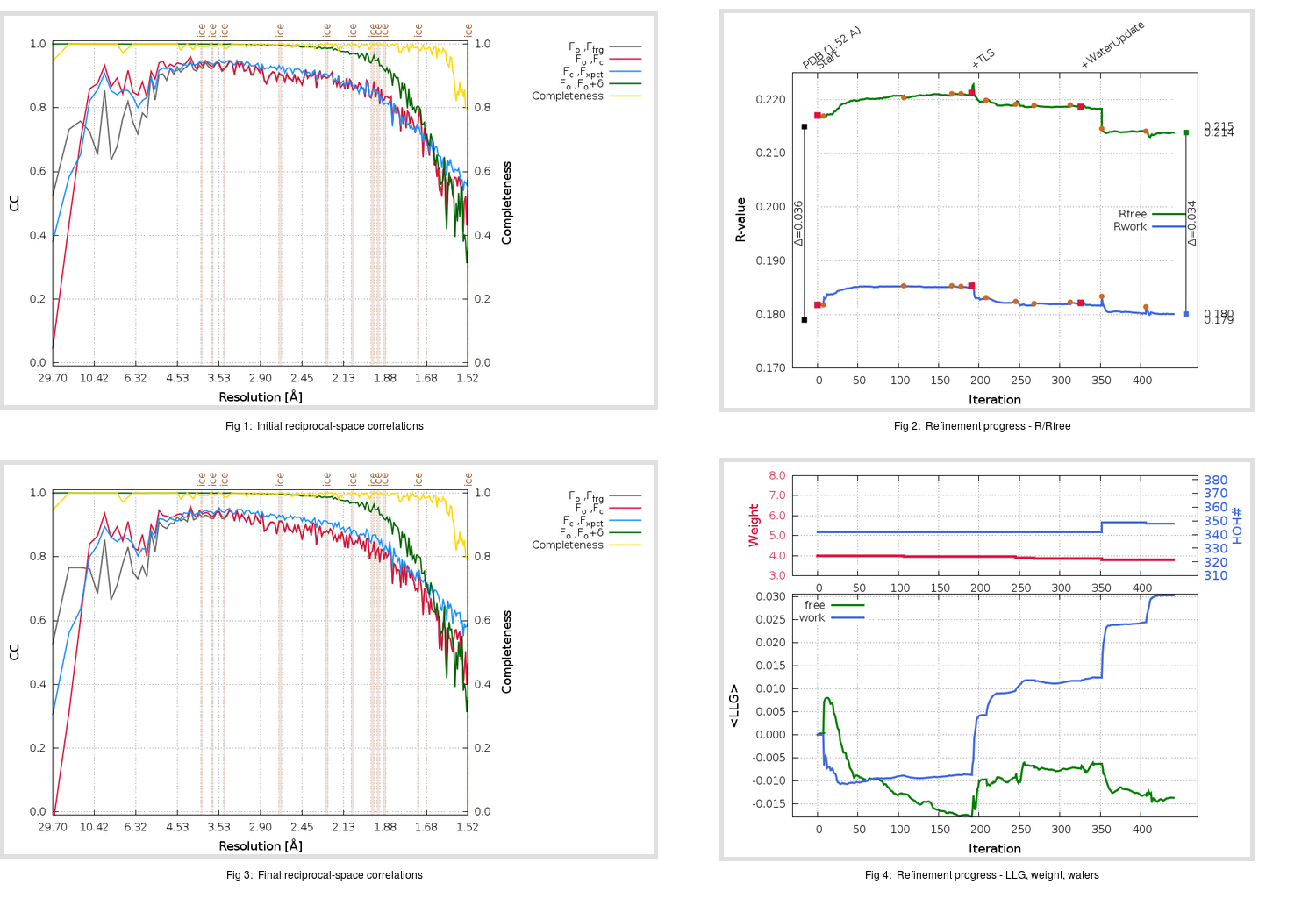Content:

## Deposited

` `
 Date deposited Date data collection Resolution R, Rfree 20200315 20200305 1.52 0.1770 0.2150

Molprobity (CCP4 7.0 version) summary:

```Ramachandran outliers =   0.33 %
favored =  98.33 %
Rotamer outliers      =   0.75 %
C-beta deviations     =     0
Clashscore            =   4.19
RMS(bonds)            =   0.0132
RMS(angles)           =   1.84
MolProbity score      =   1.20
Resolution            =   1.52
R-work                =   0.1770
R-free                =   0.2150
```

```Number of waters      =   342

<B> (all atoms) =   23.49 ( sd =    9.24 ) for       2763 non-hydrogen atoms
<B>   (protein) =   21.97 ( sd =    8.25 ) for       2398 non-hydrogen atoms
<B>     (water) =   33.79 ( sd =    9.12 ) for        342 non-hydrogen atoms
<B>    (others) =   28.46 ( sd =   13.59 ) for         23 non-hydrogen atoms

B min/max       (all non-hydrogen atoms) =   11.81 /   70.99
B min/max   (protein non-hydrogen atoms) =   12.03 /   70.99
B min/max     (water non-hydrogen atoms) =   11.81 /   57.27
B min/max     (other non-hydrogen atoms) =   23.40 /   42.48
```

## BUSTER (re-)refinement

` `

Molprobity (CCP4 7.0 version) summary:

```Ramachandran outliers =   0.33 %
favored =  98.33 %
Rotamer outliers      =   1.49 %
C-beta deviations     =     0
Clashscore            =   2.09
RMS(bonds)            =   0.0113
RMS(angles)           =   1.55
MolProbity score      =   1.11
Resolution            =   1.52
R-work                =   0.1801
R-free                =   0.2138
```

```Number of waters      =   348

<B> (all atoms) =   24.67 ( sd =    8.89 ) for       2769 non-hydrogen atoms
<B>   (protein) =   22.84 ( sd =    7.01 ) for       2398 non-hydrogen atoms
<B>     (water) =   36.85 ( sd =   10.36 ) for        348 non-hydrogen atoms
<B>    (others) =   27.95 ( sd =   14.20 ) for         23 non-hydrogen atoms

B min/max       (all non-hydrogen atoms) =   11.07 /   71.01
B min/max   (protein non-hydrogen atoms) =   11.07 /   63.19
B min/max     (water non-hydrogen atoms) =   13.38 /   71.01
B min/max     (other non-hydrogen atoms) =   19.89 /   40.25
```

Refinement progression:Results:

` `
 File Remark 5RFA_aB_refine.01_03_refine.pdb.gz exact refinement commands are in header 5RFA_aB_refine.01_03_refine.mtz.gz including original deposited data and several re-refinement map coefficients 5RFA_aB_refine.01_03_BUSTER_model.cif.gz including any non-standard compound restraints 5RFA_aB_refine.01_03_BUSTER_refln.cif.gz